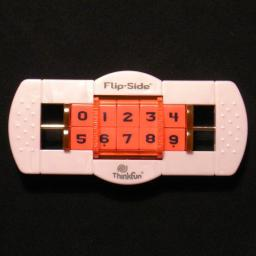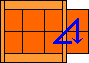# Flip-SideFlip-Side is a recent puzzle by Thinkfun. It consists of a frame containing 10 numbered blocks in two rows of five. In the centre of the frame is a turntable that holds 3 blocks from each row. When turned, the three blocks in the top row swap with the three blocks directly beneath them. Each row can slide left or right, so you can choose which three adjacent blocks in a row are flipped to the other row. The blocks are numbered, and the aim is of course to arrange them in numerical order after they have been mixed, i.e. 01234 on the top row, 56789 on the bottom row.

Several possible patterns are suggested on the Flip-Side packaging. These are shown below:

One In Front Right To Left Evens And Odds Upside Down Cycle Reverse Cycle
12345
67890
43210
98765
02468
13579
56789
01234
01234
98765
98765
01234

Flip-Side was invented by Ferdinand Lammertink, who also invented Topspin, Backspin, and Triple Cross. The puzzle's patent, US 2006/279041, was published 14 December 2006.

If your browser supports it, you can click on the link below to play with a Javascript version of Flip-Side.

## The number of positions:

There are 10 pieces, so there are at most 10! = 3,628,800 positions with both sliders centralised. All these positions can be achieved. There are 9 ways the sliders can be arranged, so if you insist on distinguishing those then there are 9 times as many possible positions.

I have calculated through all positions on my computer, and the results are in the table below. It shows that any position can be solved in at most 11 flips (8.0429 on average).

Depth    # positions
01
19
267
3493
43,469
523,385
6145,212
7714,611
81,579,683
91,075,898
1085,968
114
total3,628,800

The four hardest positions, the ones that need 11 flips, are as follows:

 5806934127 8967203514 2785603914 5846972301

Phase 1: Solve top left number, number 0.

1. Move the slider containing the 0 so that the 0 is at the left of the flip area, or if that isn't possible, slide it as far to the left as it can go.
2. Move the other slider as far to the right as it can go.
3. Flip it. This will have brought the 0 further leftwards than it was before.
4. Repeat this until the 0 is in its correct place at the top left.

Phase 2: Solve bottom left number, number 5
This is done in the same way as phase 1, except that in step b you must never put the previously solved piece 0 in the flip area. The steps are therefore:

1. Move the slider containing the 5 so that the 5 is at the left of the flip area, or if that isn't possible, slide it as far to the left as it can go.
2. Move the other slider as far to the right as it can go, but do not so far as to put a previously solved piece inside the flip area.
3. Flip it.
4. Repeat a-c this until the 5 is in its correct position.

Phase 3: Solve next two numbers, 1 and 6.
These are solved in exactly the same way, just make sure you never put any previously solved piece in the flip area.

The next section will be solved using a simple move sequence which cycles 3 pieces. Suppose you have both sliders in the central positions. Now do the following steps:
1. Move top slider left.
2. Flip.
3. Move top slider right.
4. Flip.
5. Move bottom slider left.
6. Flip.
7. Move bottom slider right.
8. Flip.This cycles around anti-clockwise the right-most two pieces of the bottom row and the right-most piece of the top row. I'll call this The Cycle. Using a fairly self-explanatory notation, this can be written as:

The Cycle:     Ul / Ur / Dl / Dr /

Note that you need not start the sequence with the sliders in the central position. One or both of the sliders may be shifted to the right beforehand, and it will still work, though the Cycle then obviously moves different pieces.

Phase 4: Solve the top row middle piece, number 2.

1. Centralise both sliders.
2. If piece 2 is not in the flip area, apply the Cycle once or twice until it is.
3. Push both sliders to the right.
4. If piece 2 is not in the flip area, apply the Cycle once or twice until it is.
5. Push both sliders completely to the left.
6. If piece 2 is in the bottom row, flip it to the top.

Phase 5: Solve the bottom row middle piece, number 7.

1. Centralise both sliders.
2. If piece 7 is not in the flip area, apply the Cycle once or twice until it is.
3. Push both sliders to the right.
4. If piece 7 is not in the flip area, apply the Cycle once or twice until it is.

Phase 6: Solve piece 3.

1. Centralise both sliders.
2. If piece 3 is in the bottom row, below its home position, then apply the Cycle.
3. Flip.
4. Apply the Cycle until piece 3 is lies in the bottom row, fourth position (i.e. next to the 2).
5. Flip.

Phase 7: Solve piece 8.

1. Centralise both sliders.
2. Apply the Cycle until piece 8 is in its correct position.

Phase 8: Solve pieces 4 and 9.

1. Centralise both sliders.
2. If 4 and 9 have to be swapped, do the following sequence:
Ul Dl / DrDr / DlDl / UrUr / UlUl / Dr DrUr / Dl / DrUr / Dl /
An easier to remember alternative is:
1. Move both sliders to the far left, and flip.
2. Move both sliders to the far right, and apply the Cycle.
3. Move both sliders to the far left, and flip.
4. Move both sliders to the far right, and apply the Cycle.
5. Move both sliders to the far left, and flip.
6. Move both sliders to the far right, and apply the Cycle.

Note: I can never remember a move sequence like the one in the last phase. Its use can be avoided however, by examining the permutation parity. Every flip changes the permutation parity. The parity is not changed by the phases 5-8 (they all use an even number of flips) but we can set the correct parity in phase 4.

Below is an alternative to phase 4, which puts piece 2 correct and ensures the permutation has the correct parity, thus avoiding phase 8. If you have arrived at phase 8 and find you need to make a single swap, then you can fix this by doing the moves in step b of the alternative phase 4 below, and then doing phases 5-7 again.

Alternative Phase 4: Solve the top row middle piece, number 2, and fix parity.

1. If piece 2 is in the middle of the bottom row, then check the permutation parity and do:
Even parity: Move both sliders to the far right, and apply the Cycle. The parity remains even.
Odd parity: Move both sliders to the far left, then flip. Piece 2 is solved, and continue with phase 5.
2. If piece 2 is in the middle of the top row, then check the permutation parity and do:
Even parity: Do nothing. Piece 2 is solved, and continue with phase 5.
Odd parity:
1. Move both sliders to the far left, and flip.
2. Move both sliders to the far right, and apply the Cycle.
The parity is now even.
3. Centralise both sliders.
4. If piece 2 is not in the flip area, apply the Cycle once or twice until it is.
5. Push both sliders to the far left.
6. Check the parity. If the parity is even, then flip to make the parity odd.
7. Push both sliders to the far right.
8. Apply the Cycle once or twice until 2 is in the middle of the bottom row.
9. Push both sliders completely to the left.
10. Flip to bring piece 2 to the top, into its correct position.

Nice patterns: